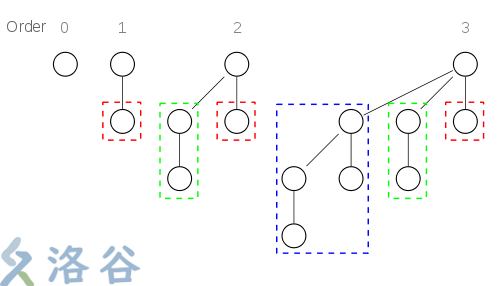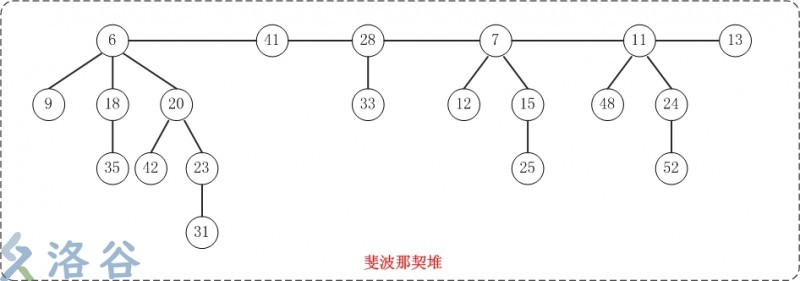# 前言

upd（2019/9/20）：修改逻辑和讲解顺序，删除不完全证明。由于该文章是一个半月以前写成的（那时候我比现在菜很多，文风也更加跳脱），并且是学习笔记性质（即边学习边写文），难免有逻辑混乱或者不当之处。敬请指出。

# 二项堆

1. 你手上有一个堆，有一个节点。
2. 有人给了你一个节点，你将两个节点合在了一起。
3. 又有人给你了两个节点，你直接找到一个小的根，一并挂上去。
4. 这回有人给了你四个节点，你还是在这两个各有四个节点的根里面找到一个最小的根，然后一股脑全挂上去。
5. 那人给了你八个节点，你厌烦了，把树甩他脸上就走人了，继续看博客。• 第$k$个二项树有$2^k$个结点（请再次看一遍扔树过程）
• 第$n$个二项树中深度为$k$的结点有$C_n^k$个（命名由来，数数就知道了，虽然没啥用）
• 可以在$\Theta(1)$的时间内合并两个相同的满足堆性质的二项树

$$\text{我们似乎搞不到一个有任意节点的二项树}$$

• 最多由$\Theta(\log n)$个满足堆性质的二项树组成
• 相同大小的二项树只有一个

## 删除一个最小数

1. 找到那个最小数（肯定是某个二项堆的根节点）。
2. 暴力去掉，把子节点都拆出来倒一起。
3. 倒出来的子节点当做一个新堆与原堆合并。

# 列表比较

emmmm……这张表有点单调QwQ。

# 斐波那契堆## 插入

$$\text{将新结点视为一个只有一个结点的堆，执行堆的合并。}$$

# 时间复杂度证明

#### 插入/合并

$\Theta(1)$

#### 查找最小数

$\Theta(1)$

#### 删除最小数

$$F_{k+1}=F_k+F_{k-1}$$

$$f(i)\geq Fib(i)$$

$$f(i)\geq Fib(i) = \frac{(\frac{1+\sqrt{5}}{2})^n-(\frac{1-\sqrt{5}}{2})^n}{\sqrt{5}}$$

# 分析&应用

Lazy algorithm能够加快运行效率的本质是因为多个相同操作可以合并，或者可以避免建立结构之后再反复调整，进一步的本质是去时序化。例如线段树的区间加，就可以将两次区间加堆到一起完成。而斐波那契堆的lazy algorithm可以减少的重复运算主要是在调整节点权值的时候进行的。如果先建立堆结构再调整节点权值的话，需要对堆结构进行维护，斐波那契堆则避免了对堆结构的维护。

Dijkstra啊！